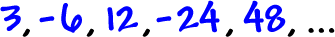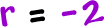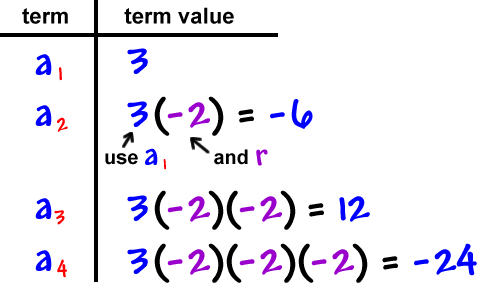OK, so how can we find that magical nth term for a geometric sequence?  (Remember that this will get you that sigma notation to generate the series for these.)

Let's do one the long way and figure out how the formula works for these.

Let's find the 50th term and the nth term of this geometric sequenceFirst we need the ratio:Now, let's do the table thang!This is getting messy!  Let's streamline the notation so we can nail the pattern.